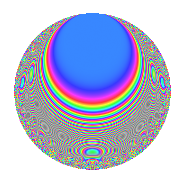Properties

 Label 1336.2.aLevel 1336 Weight 2 Character orbit a Rep. character $$\chi_{1336}(1,\cdot)$$ Character field $$\Q$$ Dimension 42 Newforms 5 Sturm bound 336 Trace bound 3

Related objects

Defining parameters

 Level: $$N$$ = $$1336 = 2^{3} \cdot 167$$ Weight: $$k$$ = $$2$$ Character orbit: $$[\chi]$$ = 1336.a (trivial) Character field: $$\Q$$ Newforms: $$5$$ Sturm bound: $$336$$ Trace bound: $$3$$ Distinguishing $$T_p$$: $$3$$

Dimensions

The following table gives the dimensions of various subspaces of $$M_{2}(\Gamma_0(1336))$$.

Total New Old
Modular forms 172 42 130
Cusp forms 165 42 123
Eisenstein series 7 0 7

The following table gives the dimensions of the cuspidal new subspaces with specified eigenvalues for the Atkin-Lehner operators and the Fricke involution.

$$2$$$$167$$FrickeDim.
$$+$$$$+$$$$+$$$$9$$
$$+$$$$-$$$$-$$$$12$$
$$-$$$$+$$$$-$$$$12$$
$$-$$$$-$$$$+$$$$9$$
Plus space$$+$$$$18$$
Minus space$$-$$$$24$$

Trace form

 $$42q$$ $$\mathstrut -\mathstrut 2q^{5}$$ $$\mathstrut +\mathstrut 38q^{9}$$ $$\mathstrut +\mathstrut O(q^{10})$$ $$42q$$ $$\mathstrut -\mathstrut 2q^{5}$$ $$\mathstrut +\mathstrut 38q^{9}$$ $$\mathstrut -\mathstrut 4q^{11}$$ $$\mathstrut -\mathstrut 2q^{13}$$ $$\mathstrut -\mathstrut 8q^{17}$$ $$\mathstrut -\mathstrut 4q^{19}$$ $$\mathstrut +\mathstrut 42q^{25}$$ $$\mathstrut -\mathstrut 12q^{27}$$ $$\mathstrut +\mathstrut 8q^{29}$$ $$\mathstrut -\mathstrut 8q^{31}$$ $$\mathstrut -\mathstrut 8q^{33}$$ $$\mathstrut -\mathstrut 12q^{35}$$ $$\mathstrut -\mathstrut 2q^{37}$$ $$\mathstrut +\mathstrut 16q^{39}$$ $$\mathstrut -\mathstrut 4q^{41}$$ $$\mathstrut -\mathstrut 2q^{43}$$ $$\mathstrut -\mathstrut 10q^{45}$$ $$\mathstrut +\mathstrut 4q^{47}$$ $$\mathstrut +\mathstrut 38q^{49}$$ $$\mathstrut +\mathstrut 24q^{51}$$ $$\mathstrut -\mathstrut 6q^{53}$$ $$\mathstrut +\mathstrut 8q^{55}$$ $$\mathstrut -\mathstrut 28q^{57}$$ $$\mathstrut +\mathstrut 18q^{59}$$ $$\mathstrut +\mathstrut 36q^{63}$$ $$\mathstrut +\mathstrut 12q^{65}$$ $$\mathstrut +\mathstrut 30q^{67}$$ $$\mathstrut +\mathstrut 12q^{69}$$ $$\mathstrut +\mathstrut 20q^{71}$$ $$\mathstrut -\mathstrut 24q^{73}$$ $$\mathstrut +\mathstrut 4q^{75}$$ $$\mathstrut +\mathstrut 8q^{77}$$ $$\mathstrut -\mathstrut 12q^{79}$$ $$\mathstrut +\mathstrut 34q^{81}$$ $$\mathstrut -\mathstrut 6q^{83}$$ $$\mathstrut -\mathstrut 16q^{85}$$ $$\mathstrut +\mathstrut 32q^{87}$$ $$\mathstrut +\mathstrut 4q^{89}$$ $$\mathstrut -\mathstrut 20q^{91}$$ $$\mathstrut +\mathstrut 24q^{93}$$ $$\mathstrut +\mathstrut 24q^{95}$$ $$\mathstrut +\mathstrut 20q^{99}$$ $$\mathstrut +\mathstrut O(q^{100})$$

Decomposition of $$S_{2}^{\mathrm{new}}(\Gamma_0(1336))$$ into irreducible Hecke orbits

Label Dim. $$A$$ Field CM Traces A-L signs $q$-expansion
$$a_2$$ $$a_3$$ $$a_5$$ $$a_7$$ 2 167
1336.2.a.a $$2$$ $$10.668$$ $$\Q(\sqrt{5})$$ None $$0$$ $$3$$ $$0$$ $$-7$$ $$+$$ $$+$$ $$q+(1+\beta )q^{3}+(1-2\beta )q^{5}+(-4+\beta )q^{7}+\cdots$$
1336.2.a.b $$7$$ $$10.668$$ $$\mathbb{Q}[x]/(x^{7} - \cdots)$$ None $$0$$ $$-6$$ $$0$$ $$-1$$ $$+$$ $$+$$ $$q+(-1+\beta _{1})q^{3}+(-\beta _{4}+\beta _{5})q^{5}+(-1+\cdots)q^{7}+\cdots$$
1336.2.a.c $$9$$ $$10.668$$ $$\mathbb{Q}[x]/(x^{9} - \cdots)$$ None $$0$$ $$-1$$ $$-8$$ $$2$$ $$-$$ $$-$$ $$q-\beta _{1}q^{3}+(-1-\beta _{2}-\beta _{8})q^{5}+(\beta _{1}+\cdots)q^{7}+\cdots$$
1336.2.a.d $$12$$ $$10.668$$ $$\mathbb{Q}[x]/(x^{12} - \cdots)$$ None $$0$$ $$-1$$ $$8$$ $$-4$$ $$-$$ $$+$$ $$q-\beta _{1}q^{3}+(1-\beta _{9})q^{5}+\beta _{11}q^{7}+(2+\cdots)q^{9}+\cdots$$
1336.2.a.e $$12$$ $$10.668$$ $$\mathbb{Q}[x]/(x^{12} - \cdots)$$ None $$0$$ $$5$$ $$-2$$ $$10$$ $$+$$ $$-$$ $$q+\beta _{1}q^{3}+\beta _{9}q^{5}+(1+\beta _{11})q^{7}+(2+\cdots)q^{9}+\cdots$$

Decomposition of $$S_{2}^{\mathrm{old}}(\Gamma_0(1336))$$ into lower level spaces

$$S_{2}^{\mathrm{old}}(\Gamma_0(1336)) \cong$$ $$S_{2}^{\mathrm{new}}(\Gamma_0(167))$$$$^{\oplus 4}$$$$\oplus$$$$S_{2}^{\mathrm{new}}(\Gamma_0(334))$$$$^{\oplus 3}$$$$\oplus$$$$S_{2}^{\mathrm{new}}(\Gamma_0(668))$$$$^{\oplus 2}$$